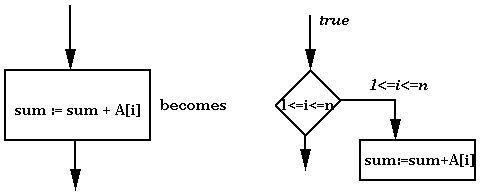## Proof-Carrying Code

Lecturer: Professor Fred B. Schneider

Lecture notes by Lynette I. Millett

In SFI, our approach was to take the code, fix it, and then run the result. As long as we trust the "fixing" process, then we trust that the security policy we're interested in will not be violated. There is another approach, which is to take the code, check it, and then run the program (if the checker says the program is ok.) Here, the checker is part of the trusted computing base (TCB). In this scheme, properties of the program are unchanged.

The question is: How can we check the program? This is equivalent to proving a theorem, namely: the program does not read/write/jump outside of its LFD. However, we don't want the operating system to have to construct this proof for each program. Moreover, some things are impossible to prove (e.g. we can't prove a program does not halt). The solution is to expect the program to be accompanied by its proof. Now, we only need check that the proof is valid, and that is a reasonable task for an operating system's loader. We demonstrate how this can be done for a simple flow-chart programming language.

Consider the following program: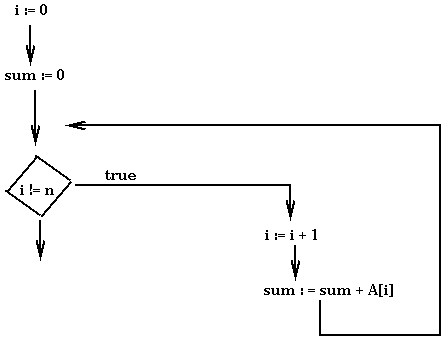Suppose we would like to prove that access to array A[1..n] is always in bounds. We need to show that whenever a reference is made to array element A[i] that i is between 1 and n. We will annotate the flowcharts with predicates. There are two conditions on these predicates:
• The in-edge to any statement referencing an array element A[i] is labelled by a predicate that implies 1 <= i <= n.
• If execution is started on an edge and the corresponding predicate holds, then as each successive edge is reached, the predicate labelling that edge will hold as well.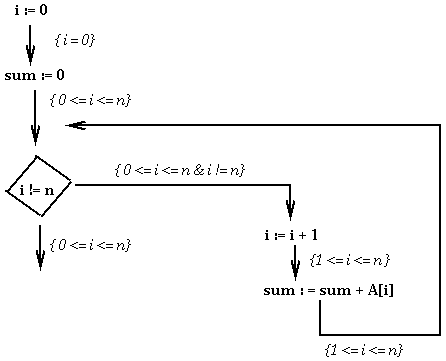In the example, the annotations constitute a proof that all accesses to array A are within range. We now discuss how to check such a proof. This entire method is referred to as proof-carrying code (PCC) because we transmit a program with its proof to a checker that then decides whether to run it or not. How the checker works depends on the language used. We will consider proofs at the level of assembly language. In this scenario, a proof can be decomposed into two kinds of elements: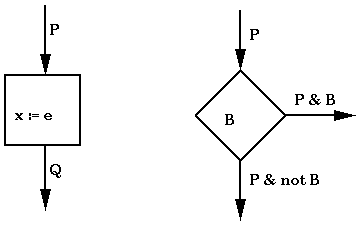If we check each piece, then the proof is checked.

Let's consider the decision box. In order to check this, we need to check that the 'B' on the out edges is equivalent to the 'B' in the box. A more general case would be: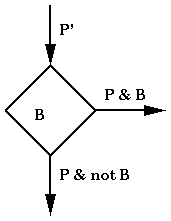To determine that this is correct we need to check that P' implies P.

For the assignment box, we need to check that P implies Qex, where Qex is defined to be Q with all instances of x replaced by e. As an example, consider the following: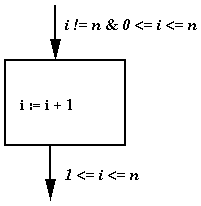We need to show that i != n & 0 <= i <= n implies (1 <= i <=n)i+1i. Note that checking proofs involves only syntactic substitution and checking strengthenings. In fact, a proof checker as described can be written in roughly 200 lines of C, which makes for a small TCB. It also happens that for policies that SFI implements, compilers can generate the needed proofs.

We now consider the relationship between SFI and PCC. Suppose we are given a program. After running it through SFI we obtain a program that has a lot of additional code. If we now put this transformed program through an optimizer, the optimizer will likely eliminate some of the additional code. In order to trust the optimized SFI'd code, the optimizer now needs to be a part of the TCB. If the optimizer can provide a narrative about what it did to the program, then the optimizer need no longer be part of the TCB. Instead we can add to the TCB a checker that evaluates the narrative to make sure the optimizer only removed gratuitous checks. The narrative is, in essence, a proof. PCC and SFI are clearly related. The former does analysis and the latter adds instructions to the program. SFI provides a program that is very easy to check the proof for.